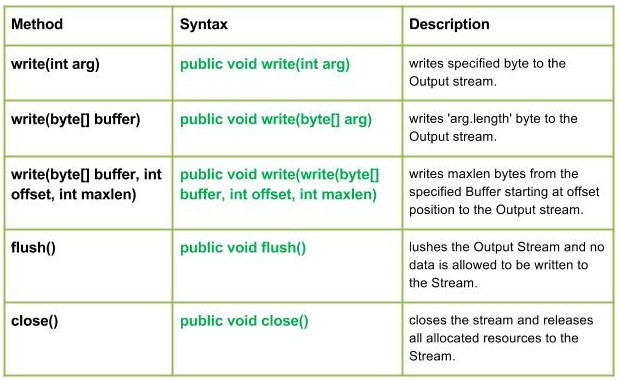Open in App
Not now

# Java.io.FilterOutputStream Class in Java

• Last Updated : 29 Oct, 2022

java.io.FilterInputStream Class in JavaJava.io.FilterOutputStream class is the superclass of all those classes which filters output streams. The write() method of FilterOutputStream Class filters the data and write it to the underlying stream, filtering which is done depending on the Streams.

Declaration :

```public class FilterOutputStream
extends OutputStream```

Constructors :

• FilterOutputStream(OutputStream geekout) : Creates an output stream filter.

Methods:

• write(int arg) : java.io.FilterOutputStream.write(int arg) writes specified byte to the Output stream.
Syntax :
```public void write(int arg)
Parameters :
arg : Source Bytes
Return  :
void
Exception :
In case any I/O error occurs.```
• Implementation :

## Java

 `// Java program illustrating the working of work(int arg)``// method``import` `java.io.*;``import` `java.lang.*;` `public` `class` `NewClass``{``    ``public` `static` `void` `main(String[] args) ``throws` `IOException``    ``{``        ``// OutputStream, FileInputStream & FilterOutputStream``        ``// initially null``        ``OutputStream geek_out = ``null``;``        ``FilterOutputStream geek_filter = ``null``;` `        ``// FileInputStream used here``        ``FileInputStream geekinput = ``null``;` `        ``char` `c;``        ``int` `a;``        ``try``        ``{``            ``// create output streams``            ``geek_out = ``new` `FileOutputStream(``"GEEKS.txt"``);``            ``geek_filter = ``new` `FilterOutputStream(geek_out);` `            ``// write(int arg) : Used to write 'M' in the file``            ``// - "ABC.txt"``            ``geek_filter.write(``77``);` `            ``// Flushes the Output Stream``            ``geek_filter.flush();` `            ``// Creating Input Stream``            ``geekinput = ``new` `FileInputStream(``"GEEKS.txt"``);` `            ``// read() method of FileInputStream :``            ``// reading the bytes and converting next bytes to int``            ``a = geekinput.read();` `            ``/* Since, read() converts bytes to int, so we``               ``convert int to char for our program output*/``            ``c = (``char``)a;` `            ``// print character``            ``System.out.println(``"Character written by"` `+``                              ``" FilterOutputStream : "` `+ c);` `        ``}``        ``catch``(IOException except)``        ``{``            ``// if any I/O error occurs``            ``System.out.print(``"Write Not working properly"``);``        ``}``        ``finally``{` `            ``// releases any system resources associated with``            ``// the stream``            ``if` `(geek_out != ``null``)``                ``geek_out.close();``            ``if` `(geek_filter != ``null``)``                ``geek_filter.close();``        ``}``    ``}``}`

• Note :
In the program I have used GEEKS.txt file, the program will create a new file of the name given in the code and write in it.
Output :
`Character written by FilterOutputStream : M`
• write(byte[] buffer) : java.io.FilterOutputStream.write(byte[] buffer) writes ‘arg.length’ byte to the Output stream.
Syntax :
```public void write(byte[] arg)
Parameters :
buffer : Source Buffer to be written to the Output Stream
Return  :
void
Exception :
In case any I/O error occurs.```
• Implementation :

## Java

 `// Java program illustrating the working of work(byte``// buffer) method``import` `java.io.*;``import` `java.lang.*;` `public` `class` `NewClass``{``    ``public` `static` `void` `main(String[] args) ``throws` `IOException``    ``{``        ``// OutputStream, FileInputStream & FilterOutputStream``        ``// initially null``        ``OutputStream geek_out = ``null``;``        ``FilterOutputStream geek_filter = ``null``;` `        ``// FileInputStream used here``        ``FileInputStream geekinput = ``null``;` `        ``byte``[] buffer = {``77``, ``79``, ``72``, ``73``, ``84``};``        ``char` `c;``        ``int` `a;``        ``try``        ``{``         ``// create output streams``         ``geek_out = ``new` `FileOutputStream(``"ABC.txt"``);``         ``geek_filter = ``new` `FilterOutputStream(geek_out);` `         ``// writes buffer to the output stream``         ``geek_filter.write(buffer);` `         ``// forces byte contents to written out to the stream``         ``geek_filter.flush();` `         ``// create input streams``         ``geekinput = ``new` `FileInputStream(``"ABC.txt"``);` `         ``while` `((a=geekinput.read())!=-``1``)``         ``{``            ``// converts integer to the character``            ``c = (``char``)a;` `            ``// prints``            ``System.out.print(c);``         ``}``        ``}``        ``catch``(IOException except)``        ``{``            ``// if any I/O error occurs``            ``System.out.print(``"Write Not working properly"``);``        ``}``        ``finally``        ``{``            ``// releases any system resources associated``            ``// with the stream``            ``if` `(geek_out != ``null``)``                ``geek_out.close();``            ``if` `(geek_filter != ``null``)``                ``geek_filter.close();``        ``}``    ``}``}`

• Note :
In the program I have use GEEKS.txt file, the program will create a new file of the name given in the code and write in it.

Output :

`MOHIT`
• write(byte[] buffer, int offset, int maxlen) : java.io.FilterOutputStream.write(byte[] buffer, int offset, int maxlen) writes maxlen bytes from the specified Buffer starting at offset position to the Output stream.

Syntax :

```public void write(write(byte[] buffer, int offset, int maxlen)
Parameters :
buffer : Source Buffer to be written to the Output Stream
Return  :
buffer : Source Buffer to be written
offset : Starting offset
maxlen : max no. of bytes to be written to the Output Stream
Exception :
In case any I/O error occurs.```
• flush() : java.io.FilterOutputStream.flush() flushes the Output Stream and no data is allowed to be written to the Stream.
Syntax :
```public void flush()
Parameters :
------
Return  :
void
Exception :
In case any I/O error occurs.```
• close() : java.io.FilterOutputStream.close() closes the stream and releases all allocated resources to the Stream.
Syntax :
```public void close()
Parameters :
------
Return  :
void
Exception :
In case any I/O error occurs.```

Java program illustrating : write(byte[] buffer, int offset, int maxlen), flush(), close() methods

## Java

 `// Java program illustrating the working of``// write(byte[] buffer, int offset, int maxlen),``// flush(), close() method``import` `java.io.*;``import` `java.lang.*;` `public` `class` `NewClass``{``    ``public` `static` `void` `main(String[] args) ``throws` `IOException``    ``{``        ``// OutputStream, FileInputStream & FilterOutputStream``        ``// initially null``        ``OutputStream geek_out = ``null``;``        ``FilterOutputStream geek_filter = ``null``;` `        ``// FileInputStream used here``        ``FileInputStream geekinput = ``null``;` `        ``byte``[] buffer = {``65``, ``66``, ``77``, ``79``, ``72``, ``73``, ``84``};``        ``char` `c;``        ``int` `a;``        ``try``        ``{``            ``// create output streams``            ``geek_out = ``new` `FileOutputStream(``"ABC.txt"``);``            ``geek_filter = ``new` `FilterOutputStream(geek_out);` `            ``// write(byte[] buffer, int offset, int maxlen) :``            ``// writes buffer to the output stream``            ``// Here offset = 2, so it won't read first two bytes``            ``// then maxlen = 5, so it will print max of 5 characters``            ``geek_filter.write(buffer, ``2``, ``5``);` `            ``// forces byte contents to written out to the stream``            ``geek_filter.flush();` `            ``// create input streams``            ``geekinput = ``new` `FileInputStream(``"ABC.txt"``);` `            ``while` `((a = geekinput.read())!=-``1``)``            ``{``                ``// converts integer to the character``                ``c = (``char``)a;` `                ``// prints``                ``System.out.print(c);``            ``}``        ``}``        ``catch``(IOException except)``        ``{``            ``// if any I/O error occurs``            ``System.out.print(``"Write Not working properly"``);``        ``}``        ``finally``        ``{``            ``// releases any system resources associated``            ``// with the stream``            ``if` `(geek_out != ``null``)``                ``geek_out.close();``            ``if` `(geek_filter != ``null``)``                ``geek_filter.close();``        ``}``    ``}``}`

Note :
In the program I have use GEEKS.txt file, the program will create a new file of the name given in the code and write in it.

Output :

`MOHIT`

This article is contributed by Mohit Gupta 🙂. If you like GeeksforGeeks and would like to contribute, you can also write an article using write.geeksforgeeks.org or mail your article to review-team@geeksforgeeks.org. See your article appearing on the GeeksforGeeks main page and help other Geeks.